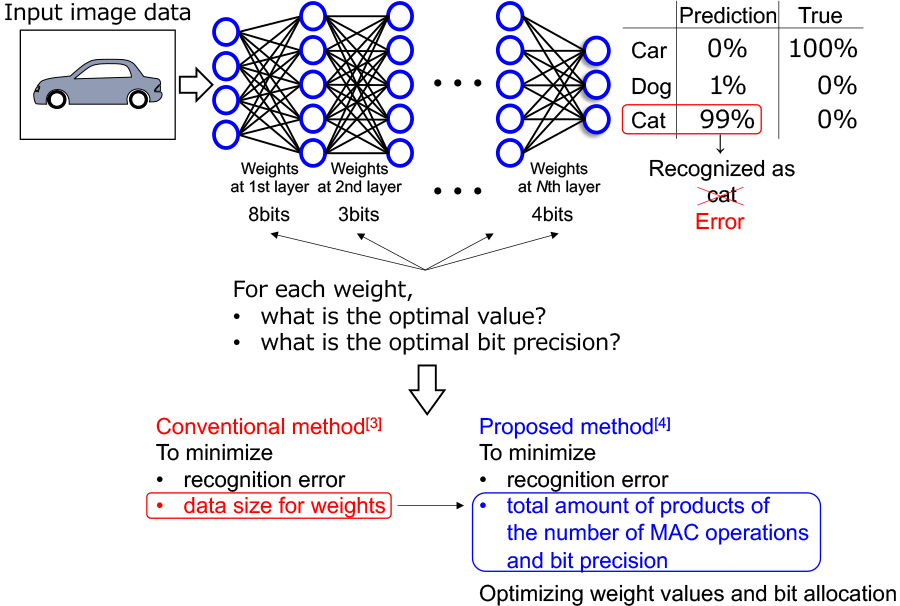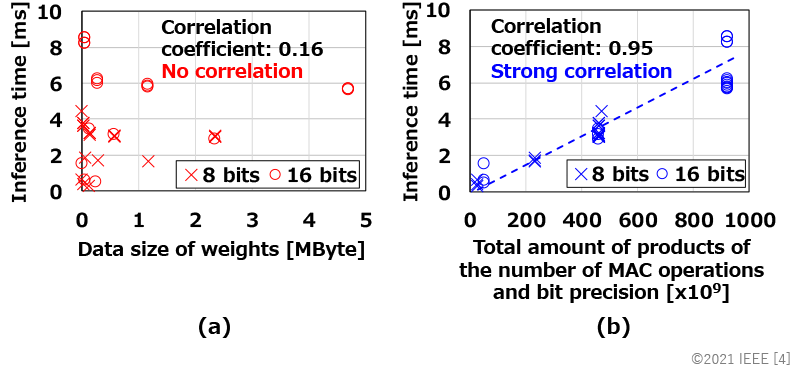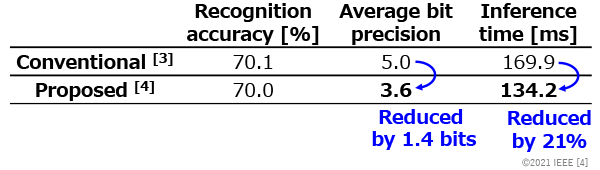# Development of quantization algorithm for accelerating deep learning inference

July 16, 2021

Deep neural networks are widely used for image recognition tasks in recent years. The process to recognize input images with deep neural networks is called inference. An inference requires millions to billions of multiply-accumulate (MAC) operations, therefore the reduction of the processing time and energy consumption is a challenge. Quantization*1 is one of the methods to reduce the processing time for inference (hereinafter referred to as inference time). We have developed not only a filter-wise quantization algorithm which optimizes the number of weight bits for each one of tens or thousands of filters on every layer but also the dedicated accelerator. With these  algorithm and hardware architecture, the inference time can be reduced while maintaining recognition accuracy. It is important to allocate the optimal number of bits to weights depending on each layer or filter (upper part of Fig. 1).

A conventional method finds out the optimal bit allocations through training. Generally, weight values are tuned iteratively to recognize input image data correctly, in other words to minimize recognition error. After training, we can obtain optimized weight values. In addition to this weight optimization, the conventional method optimizes bit allocation by tuning the bit allocation iteratively under the condition of minimizing the recognition error and data size of weights*2 (lower left of Fig. 1).Fig. 1: The difference of policy between conventional and proposed methods for optimizing weight values and bit allocation.

After the optimization, we can obtain the optimized bit allocation. This method is effective to find weight values and bit allocation which achieve better recognition accuracy with less total data size of weights. As shown in Fig. 2(a), however, there is no strong correlation between the data size of weights and inference time, and the inference time is not always reduced even if the data size of weights becomes smaller. Therefore, the conventional method is not optimal for reducing inference time.

Here, we focus on a metric which accumulates products of the number of MAC operations and bit precision. This metric includes the number of MAC operations as well as the number of bit precision, considering the impact of MAC operations to inference time. As shown in Fig. 2(b), this metric strongly correlates with inference time, and the inference time can be reduced if the metric value becomes smaller. Based on this result, we optimize weight values and bit allocation under the condition of minimizing the recognition error and the metric value (lower right of Fig. 1). Consequently, we can obtain optimized weight values and bit allocation for achieving better recognition accuracy with less inference time.Fig. 2: Correlations between inference time and (a) data size of weights/(b) total amount of products of the number of MAC operations and bit precision. The inference time of the ResNet-18*3 model is evaluated with 8 and 16 bits on NVIDIA Tesla T4 GPU.

We applied the proposed method to the optimization for weight values and bit allocation of the ResNet-18*3 model. We evaluated the recognition accuracy with the ImageNet*4 dataset and the inference time with our dedicated accelerator. As shown in Table 1, the optimized model by our proposed method achieves 70.0% recognition accuracy, which is almost the same as the optimized one by the conventional method, while reducing the average bit precision by 1.4 bits and the inference time by 21%.Table 1: Performance comparison. We evaluate optimized ResNet18*3 models on the ImageNet*4 dataset. The inference time is evaluated on the dedicated accelerator.

We presented this result at the International Symposium on Circuits and Systems 2021 (ISCAS2021).

1. Quantization: Reducing processing time and energy consumption by lowering bit precision for MAC operations.
2. Data size of weights: Data size for weight values stored in the storage. For example, if a weight has 100 elements and are stored in 8 bits, the data size is 100Byte.
3. ResNet-18: One of the deep neural networks, generally used to benchmark deep-learning for image recognition.
4. ImageNet: A large image database, generally used to benchmark image-recognition, the number of image data is over 14,000,000.

 A. Maki, D. Miyashita, K. Nakata, F. Tachibana, T. Suzuki, and J. Deguchi, "FPGA-based CNN Processor with Filter-Wise-Optimized Bit Precision," 2018 IEEE Asian Solid-State Circuits Conference (A-SSCC), pp. 47-50 (2018).
 S. Sasaki, A. Maki, D. Miyashita, and J. Deguchi, “Post Training Weight Compression with Distribution-based Filter-wise Quantization Step,” 2019 IEEE Symposium in Low-Power and High-Speed Chips (COOL CHIPS), pp. 1–3 (2019).
 S. Uhlich, L. Mauch, F. Cardinaux, K. Yoshiyama, J. A. Garcia, S. Tiedemann, T. Kemp, and A. Nakamura, “Mixed Precision DNNs: All you need is a good parametrization,” International Conference on Learning Representations (ICLR), 2020.
 K. Nakata, D. Miyashita, J. Deguchi, and R. Fujimoto, "Adaptive Quantization Method for CNN with Computational-Complexity-Aware Regularization," 2021 IEEE International Symposium on Circuits and Systems (ISCAS), pp. 1-5 (2021).

This material is a partial excerpt and a reconstruction of the reference  © 2021 IEEE.